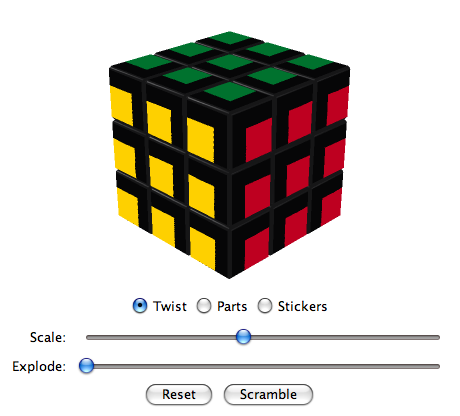# Virtual CubesActivate JavaScript to see the Virtual Cubes!

### Three Color Super Cube

The Three Color Super Cube displays only 3 colors which are shown on adjacent faces. Due to this particular color scheme and the asymmetric arangement of the stickers all pieces are distinct. The non centered stickers visualize the orientation of side parts and make this cube a Super Cube.

A Super Cube is a design variation of the Rubik's Cube which visualizes the orientation of side parts. Because of this, a Super Cube has 2048 times more positions than a regular cube (46/2 = 2048).

The layout of the Three Color Super Cube was created in 2010 by Ortwin Schenker.

#### Algorithms to twist the centers

Algorithm
(U R U R')5 (20 ltm, 20 ftm, 20 qtm)
Permutation (++u)

Algorithm M. B. Thistlethwaite
(U · R L · U2 · R' L')2 (12* ltm, 12* ftm, 14 qtm)
Permutation (++u)

Algorithm
(U' R2 U2 R' U2 R2)3 (18 ltm, 18 ftm, 30 qtm)
Permutation (+u) (+r)

Algorithm Herbert Kociemba 2008
D' R · U D · R2 U2 MF MD B MD' MF' U2 R2 U' (14* ltm, 18 ftm, 22 qtm)
Permutation (+u) (+r)

Algorithm
(MR' MD' MR) U' (MR' MD MR) U (8* ltm, 14* ftm, 14* qtm)
Permutation (+u) (-r)

Algorithm
(MR' MD' MR) U2 (MR' MD MR) U2 (8* ltm, 14 ftm, 16* qtm)
Permutation (++u) (++r)

Algorithm
(MR' MD2 MR) U' (MR' MD2 MR) U (8* ltm, 14* ftm, 18* qtm)
Permutation (+u) (-d)

Algorithm
(MR' MD2 MR U2)2 (8* ltm, 14 ftm, 20 qtm)
Permutation (++u) (++d)

Algorithm Herbert Kociemba 2008
CD' · MR MD MR' D MR MF MR' F' MD MF' MD' F U' (13* ltm, 22 ftm, 22* qtm)
Permutation (+u) (+r) (+f) (+d) (-l) (+b)

Notation